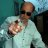# Get price difference between signals

J

#### jox51

##### Member
2019 Donor
VIP
So, I have an indicator I am working on that generates buy/sell signals. I am try to calculate the price change or difference between each buy signal and summing the result. I have been racking my brain trying to get this to work. What would be the thinkscript logic to accomplish this. So far i have this below.

bullishpb is the buysignal for example. My logic is that with fold, it will loop through each bar in the chart and if a bullishpb signal is found, then get the value of that close. When I did this, I get a 0.

def bupchg = fold b = 0 to 252 do if bullishpb is true then GetValue(close,-b) else double.nan;#### mc01439

##### Well-known member
2019 Donor
VIP
So, I have an indicator I am working on that generates buy/sell signals. I am try to calculate the price change or difference between each buy signal and summing the result. I have been racking my brain trying to get this to work. What would be the thinkscript logic to accomplish this. So far i have this below.

bullishpb is the buysignal for example. My logic is that with fold, it will loop through each bar in the chart and if a bullishpb signal is found, then get the value of that close. When I did this, I get a 0.

def bupchg = fold b = 0 to 252 do if bullishpb is true then GetValue(close,-b) else double.nan;

I think this is what you are looking for? The will give you the profit (sum) and percent positive as a label. Enter your sell and buy signals where the UPPER CASE print is. Enter the sell side first.

Code:
``````################################################################################################
input PandL_Label = Yes;

def orderDir = CompoundValue(1, if  ENTER SELL SIGNAL HERE then 1 else if ENTER BUY SIGNAL HERE then -1 else orderDir, 0);
def isOrder = orderDir crosses 0;

def orderCount = CompoundValue(1, if IsNaN(isOrder) then 0 else if isOrder then orderCount + 1 else orderCount, 0);

def noBar = IsNaN(open[-1]);

def orderPrice = if isOrder then if noBar then close else open[-1] else orderPrice;
def profitLoss = if !isOrder or orderCount == 1
then 0
else if orderDir < 0 then orderPrice - orderPrice
else if orderDir > 0 then orderPrice - orderPrice else 0;
#else if orderDir > 0 then orderPrice - orderPrice
#else if orderDir < 0 then orderPrice - orderPrice else 0;
#def profitLoss = if !isOrder or orderCount == 1 then 0 else orderPrice - orderPrice;
def profitLossSum = CompoundValue(1, if IsNaN(isOrder) then 0 else if isOrder then profitLossSum + profitLoss else profitLossSum, 0);

def orderWinners = CompoundValue(1, if IsNaN(isOrder) then orderWinners else if orderCount > 1 and profitLoss > 0 then orderWinners + 1 else orderWinners, 0);

AddLabel(PandL_Label, "[    Relative Price Oscillator  >", Color.GRAY);
AddLabel(PandL_Label, orderCount + " orders (" + AsPercent(orderWinners / orderCount) + ") | P/L " + AsDollars((profitLossSum / TickSize()) * TickValue()), if profitLossSum > 0 then Color.GREEN else if profitLossSum < 0 then Color.RED else Color.GRAY);``````

Last edited:
•jox51
J

#### jox51

##### Member
2019 Donor
VIP
Thanks @mc01439

Much appreciated.Difference between moving average and price labels Questions 10